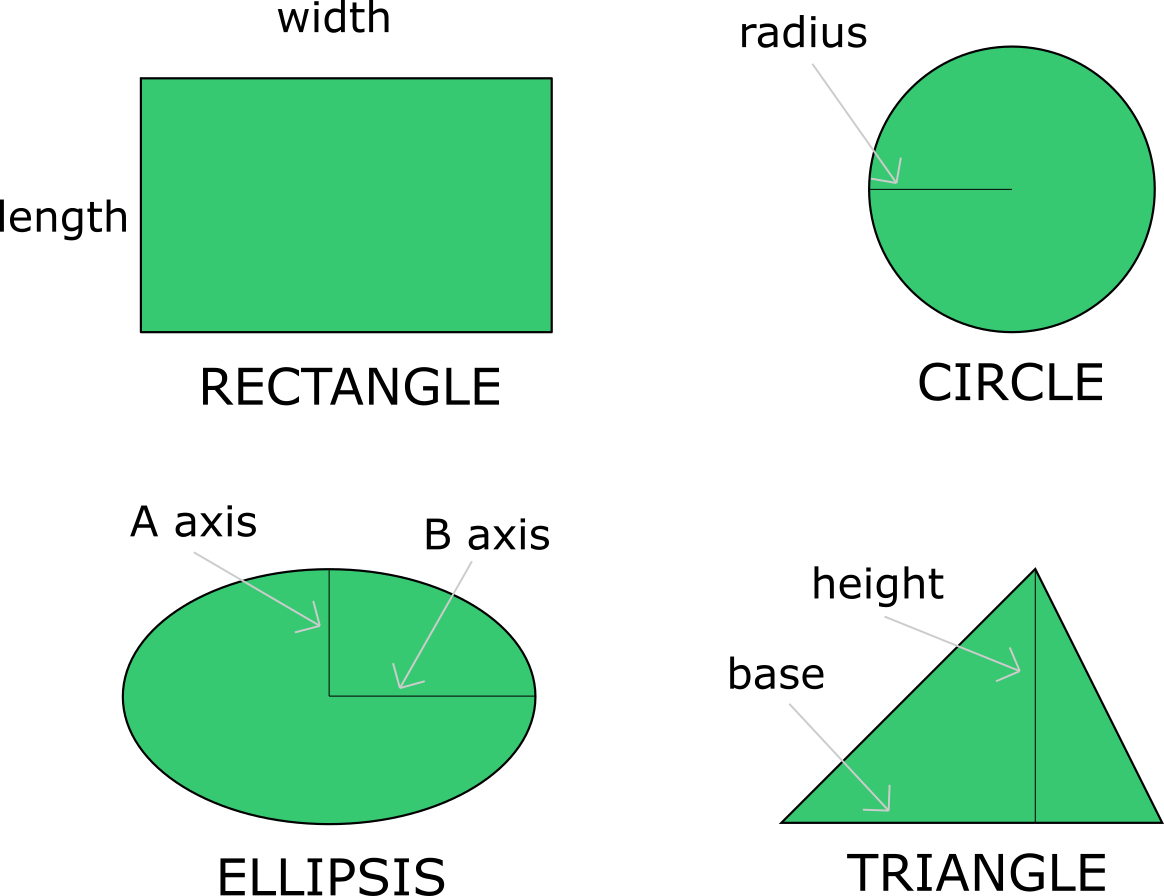Lawn area
Lawn shape
Rectangle
Width
ft
Length
ft
Area
ft²
Seeds
Coverage rate
lbs per 1000ft²
Seeds
lb

# Grass Seed Calculator

By Maria Kluziak

Whether you're tired of your garden looking so-so and want to freshen it up with a new lawn, or if you're an expert gardener and are planning some overseeding instead, don't hesitate to use this grass seed calculator to prepare your shopping! This tool will help you calculate how much grass seed do you need and plan the whole process so that your lawn grass looks its absolute best.

## How to use the grass seed calculator?

Using the grass seed calculator is easy. To calculate how much grass seed do you need for your lawn, follow these few simple steps:

1. Start by choosing the shape of your lawn and input its dimensions so that the calculator can determine its area:

• For a rectangular lawn, type in its width and length.

• For a circular lawn, type in it's radius.

• For an elliptical lawn, put in the lengths of axis A and axis B.

• For a triangular lawn, put in the lengths of the base and the height of the shape.2. To get the estimated amount of seeds you need (in pounds or kilograms, as these are the units lawn grass seeds are typically sold in), input the seed's coverage rate, and the grass seed calculator will return how much grass seed do you need.

## Using the grass seed calculator - example

Let's go through an example of a lawn grass seed calculations:

• Imagine you want a circular lawn with a radius of 50 feet. To calculate the area of the circle, you will have to use the following formula:

`circle area = π * radius²`

`area = π * (50ft)² = 7,854ft² `

• To calculate the amount of grass seeds you need, you have to know the coverage rate of your chosen species of grass. Let's say your lawn grass has a CR of 5 pounds per 1000ft². Now, let's calculate the amount of seeds:

`seeds = coverage rate * area / 1000 `

`seeds = 5 * 7,854 / 1000 = 39.35 lbs `

• Thanks to these simple calculations we now know that, to cover this area with grass, you need to buy 39.35 pounds of grass seeds.

• For the areas of the remaining shapes, the equations are as follows:

`rectangle area = width * length`

`ellipses area = axis A * axis B * π`

`triangle area = 1 /2 * height * base`

## What is the coverage rate?

The grass's coverage rate varies depending on the particular type of grass you are using. It is a measure of how much seed is needed per unit of area, for example per a thousand square feet or a hundred square meters. Don't worry if you don't know coverage rates by heart - this value is almost always written on grass seed packages. What is more, it is different depending on whether you need the seeds to create a new lawn or if you're using them for overseeding an existing one. If you are unsure of the coverage rate of the lawn grass you chose, ask a professional at the store you are buying your supplies from.

Here is a short table of the coverage rates of some of the more popular grass types:

Grass type New lawn CR Overseeding CR
Buffalo 3 lbs / 1000 ft² 1.5 lbs / 1000 ft²
Creeping Red Fescue 5 lbs / 1000 ft² 2.5 lbs / 1000 ft²
Fine Fescue 5 lbs / 1000 ft² 2.5 lbs / 1000 ft²
Kentucky Blue Grass 4 lbs / 1000 ft² 2 lbs / 1000 ft²
Tall Fescue 10 lbs / 1000 ft² 5 lbs / 1000 ft²
Maria Kluziak